#### 相似文章MetaTrader 5交易 | 26 七月 2022, 08:591 2160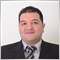### 概述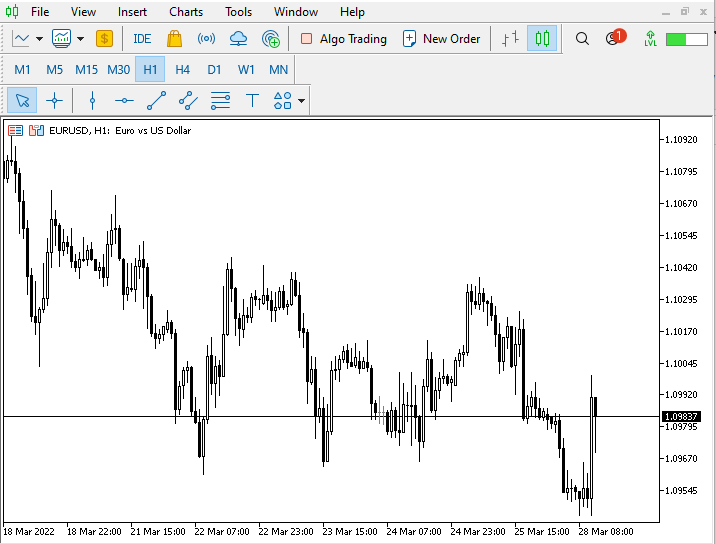• 提升或衰退（正向走势或负向走势）。
• 无提升或衰退。
• 提升或衰退。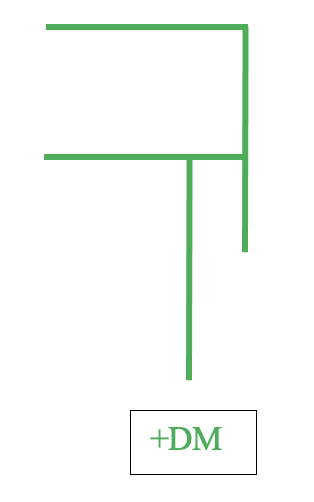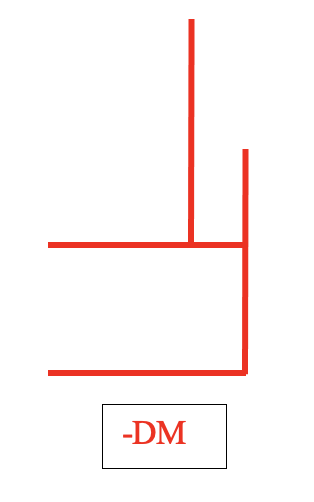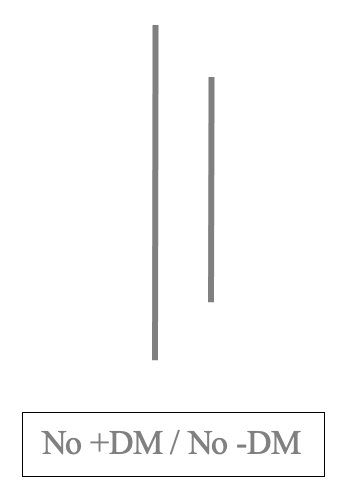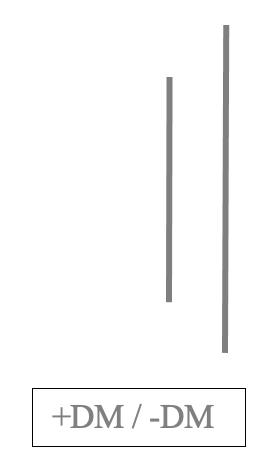1- 计算 +DM 和 -DM:

• 如果有向上走势，则 (+DM) 指正向走势。

• 如果有向下走势，则 (-DM) 指负向走势。

• 如果我们得到 +DM，那么：
+DM=n
-DM = 0
• 如果我们得到 -DM，那么：

-DM = n

+DM = 0

• 如果我们没有 (+DM 和-DM)，那么:

+DM = 0

-DM = 0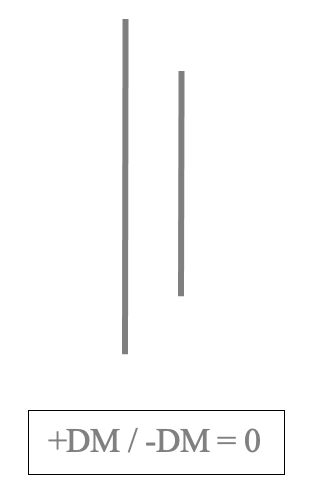• 如果我们有 (+DM 和-DM) 两者的数值，那么我们将在 +DM 和 -DM 之间进行比较，并考虑最大值，如果数值彼此相等，则忽略这些值：
+DM>-DM = 只会考虑 +DM，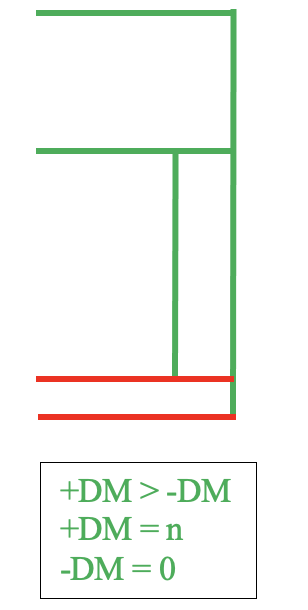+DM<-DM = 只会考虑 -DM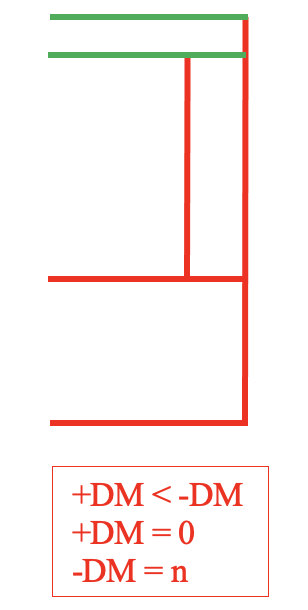+DM=-DM = 两者均被忽略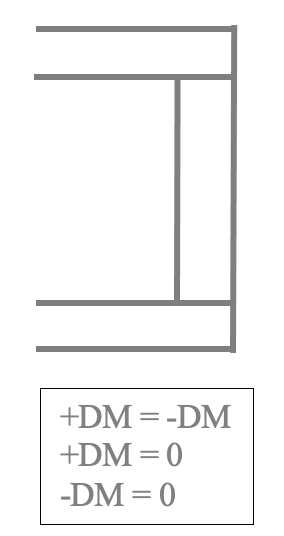2- 计算真实范围（True Range -TR):

• 当前最高价和最低价之间的差值。
• 当前最高价高与前一个收盘价之间的差值。
• 当前最低价和前一个收盘价之间的差值。
3- 求已计算出的 14 个周期的总和（+DM、-DM 和 TR）

+DM 15 = (前一 +DM - (前一 +DM/14)) + 当前 +DM

-DM 15 = (前一 -DM - (前一 -DM/14)) + 当前 -DM

TR 15 = (前一 TR - (前一 TR/14)) + 当前 TR

4- 计算 14 周期的 +DI 和 -DI
+DI 14 = 100*(+DM14/TR14)
-DI 14 = 100*(-DM14/TR14)

5- 计算 +DI 和 -DI 之间的差值

DI 14 差值 = (+DI 14) - (-DI 14)

6- 计算 +DI 和 -DI 的合计

DI 14 合计 = (+DI 14) + (-DI 14)

7- 计算 DX

DX = 100*((+DI 和 -DI 之间差值)/+DI 和 -DI 的合计))
ADX = DX 的 14 周期均值

1 110 90 100
2 140 120 130
3 160 120 140
4 150 110 130
5 140 100 130
6 150 130 140
7 170 150 160
8 180 160 170
9 170 150 155
10 170 130 140
11 180 155 160
12 190 175 180
13 220 190 190
14 230 200 200
15 215 205 210
16 200 190 200
17 195 180 190
18 195 180 185
19 210 185 195
20 220 190 200
21 233 195 208
22 246 200 216
23 258 205 223
24 271 210 231
25 283 215 238
26 296 220 246
27 308 225 253
28 321 230 261
29 333 235 268
30 346 240 276

1- 计算 +DM 和 -DM:

+DM = 当前最高价 - 前一最高价

-DM = 当前最低价 - 前一最低价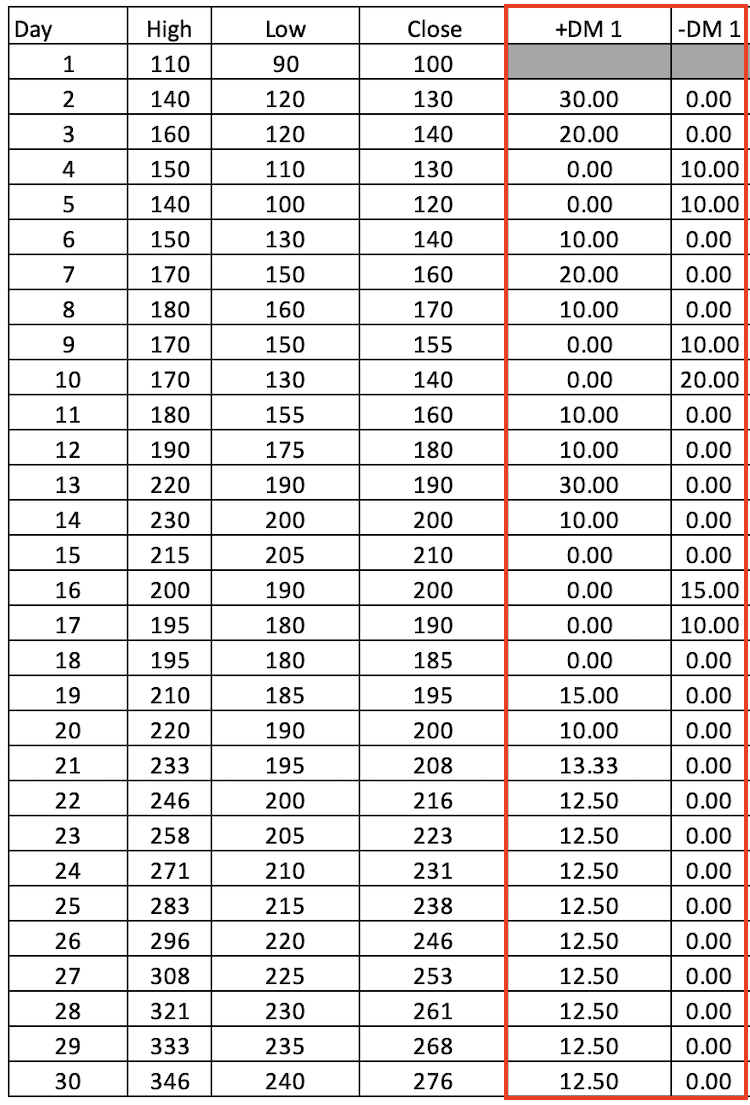2- 计算 TR:

• 当前最高价和最低价之间的差值。
• 当前最高价高与前一个收盘价之间的差值。
• 当前最低价和前一个收盘价之间的差值。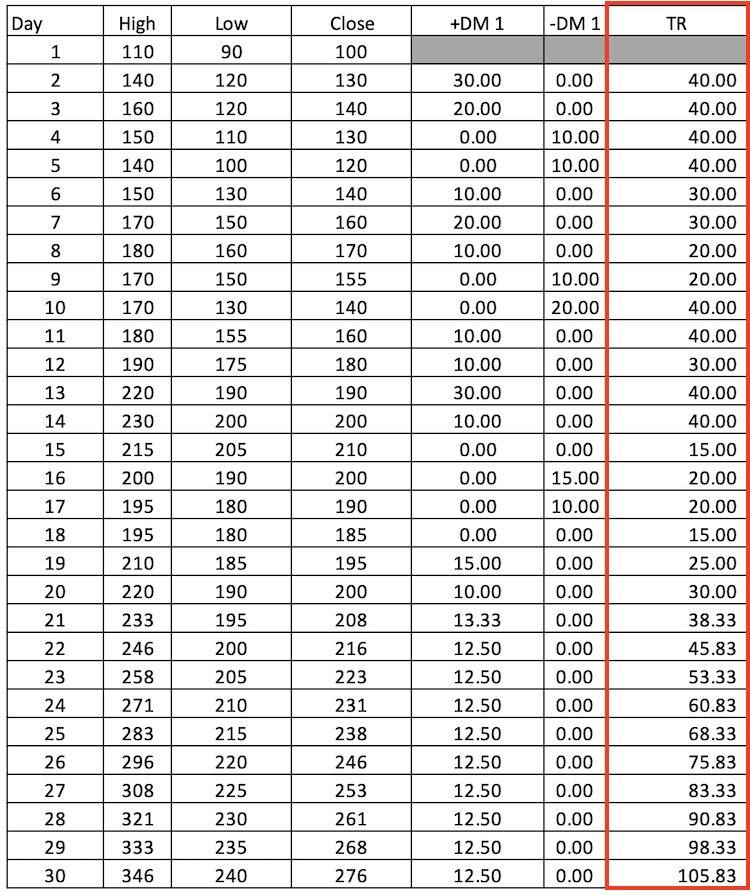3- 已计算出的 14 周期的 +DM、-DM 和 TR 求合：

+DM 14 = 合计 +DM 1(天数 2: 天数 15)

-DM 14 = 合计 -DM 1(天数 2: 天数 15)

TR 14 = 合计 TR (天数 2: 天数 15)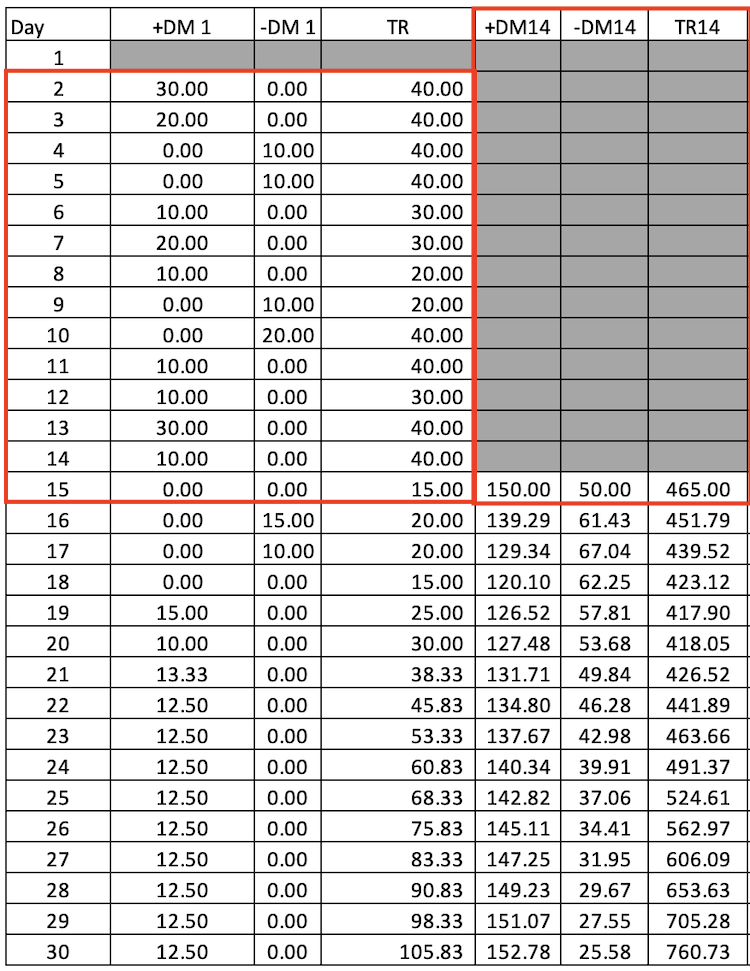4- 计算 14 周期的 +DI 和 DI，第一次计算将从第 15 天开始，因为我们需要 14 DM 来计算 14 DI：

+DI14 = 100*(+DM14/TR14)

-DI14 = 100*(-DM14/TR14)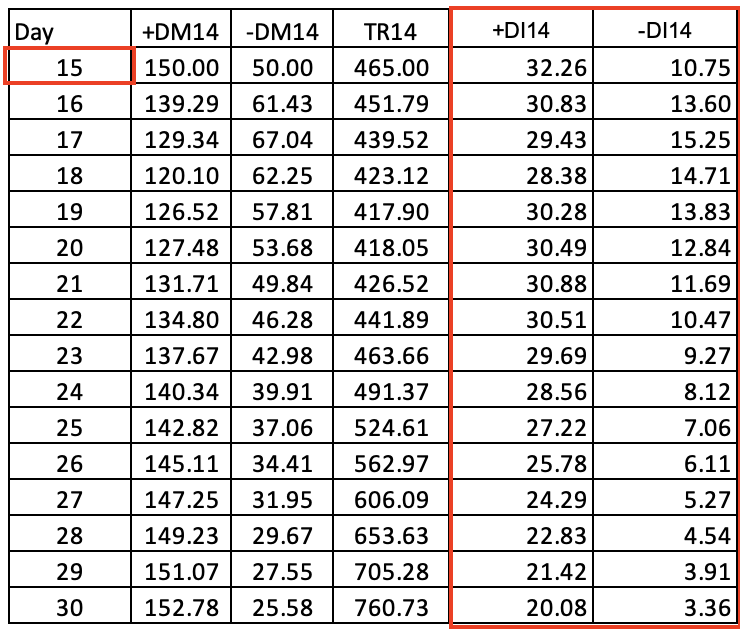5- 计算 +DI 和 -DI 之间的差值

DI 14 差值 = (+DI 14) - (-DI 14)

6- 计算 +DI 和 -DI 的合计

DI 14 合计 = (+DI 14) + (-DI 14)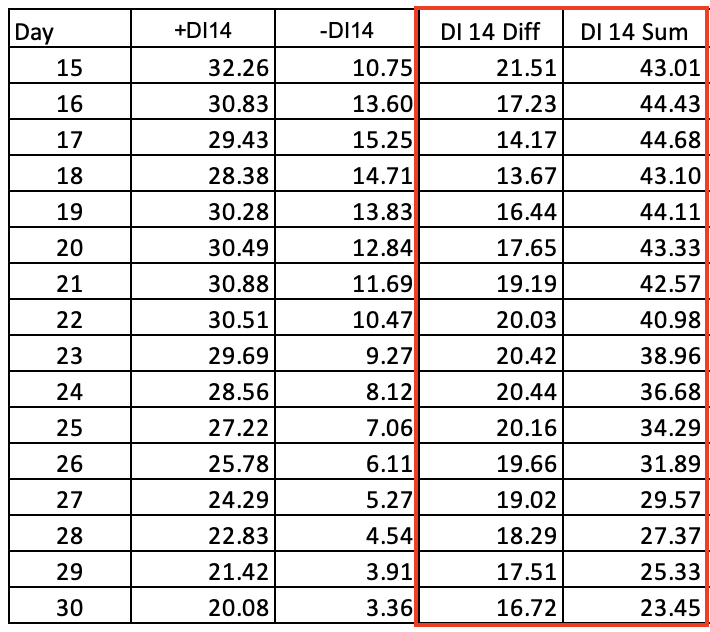7- 计算 DX:

DX = 100*((+DI 和 -DI 之间差值)/+DI 和 -DI 的合计))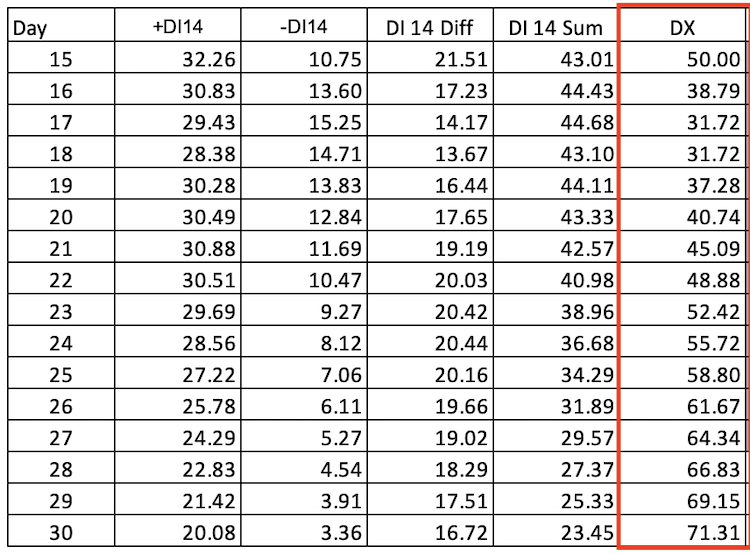ADX = 14 周期的 DX 均值 --> DI (天数 15: 天数 28) 均值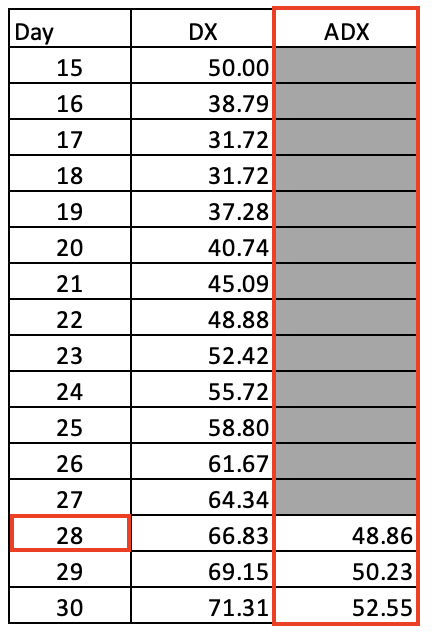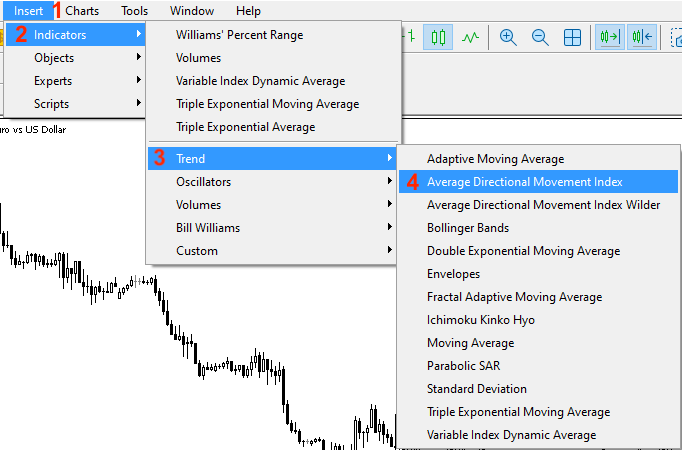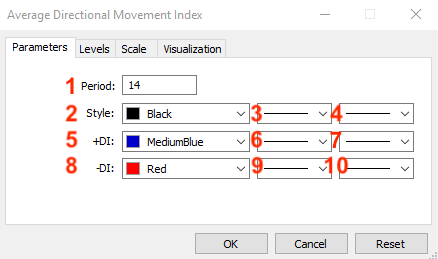1. 确定所需周期。
5. 确定 +DI 线的颜色。
6. 确定 -DI 线的颜色。
7. 确定 +DI 线的宽度。
8. 确定 -DI 线的宽度。
9. 确定 +DI 线的类型。
10. 确定 -DI 线的类型。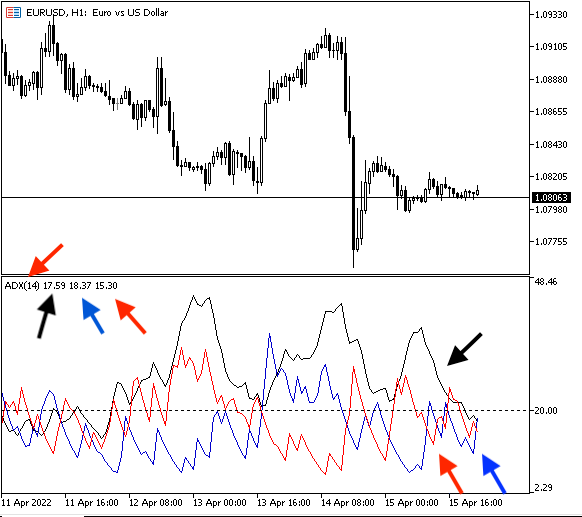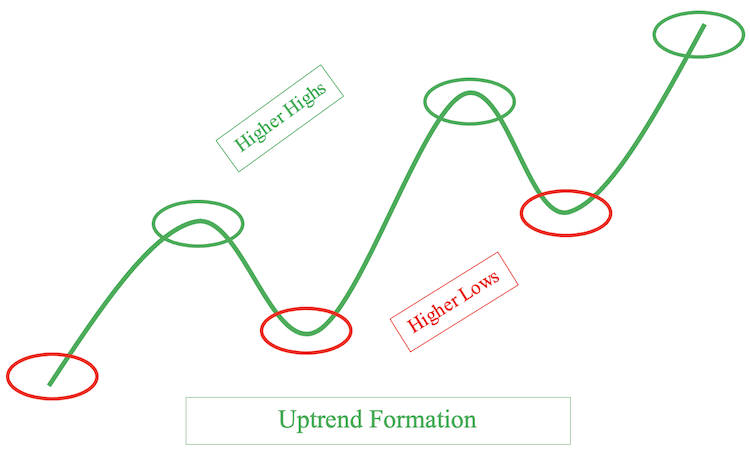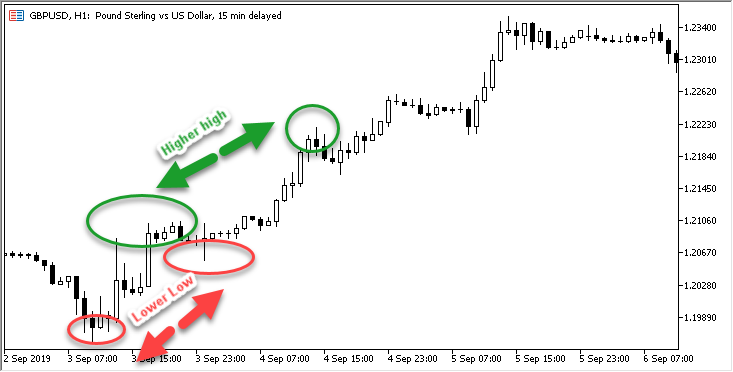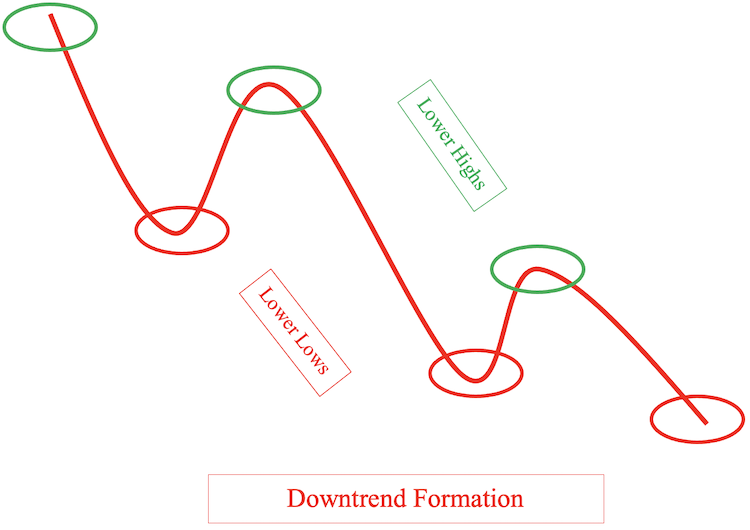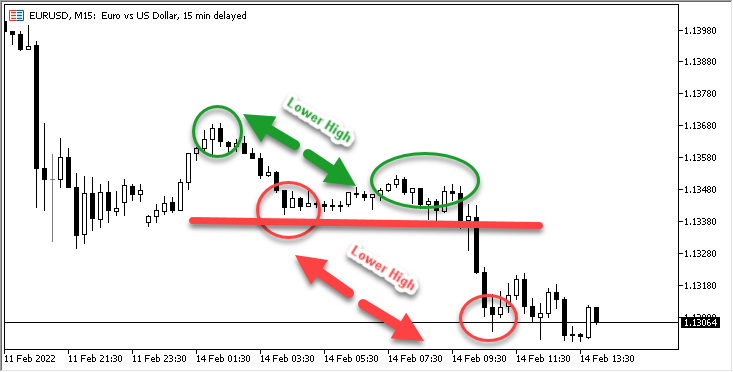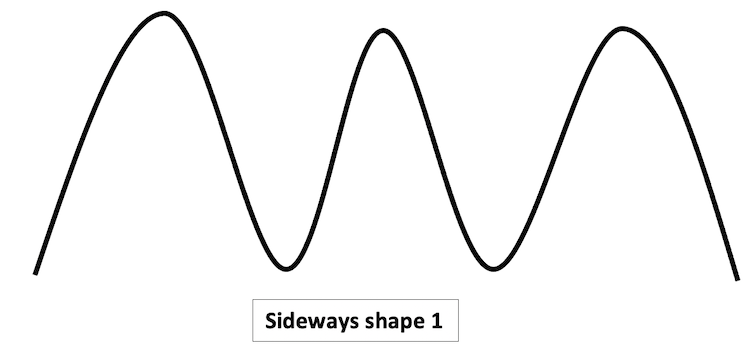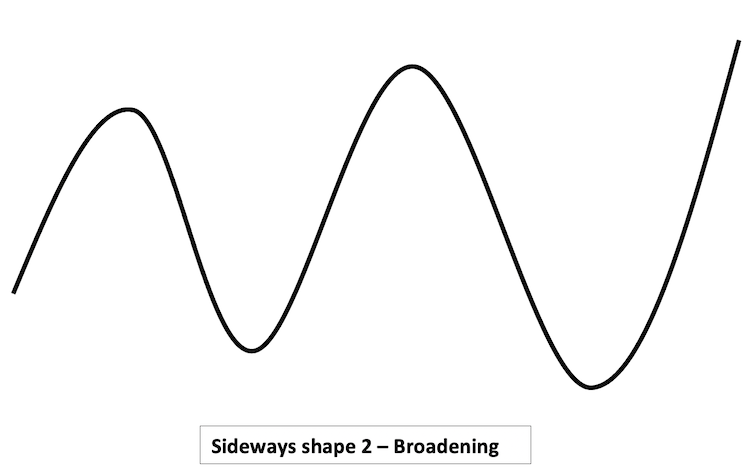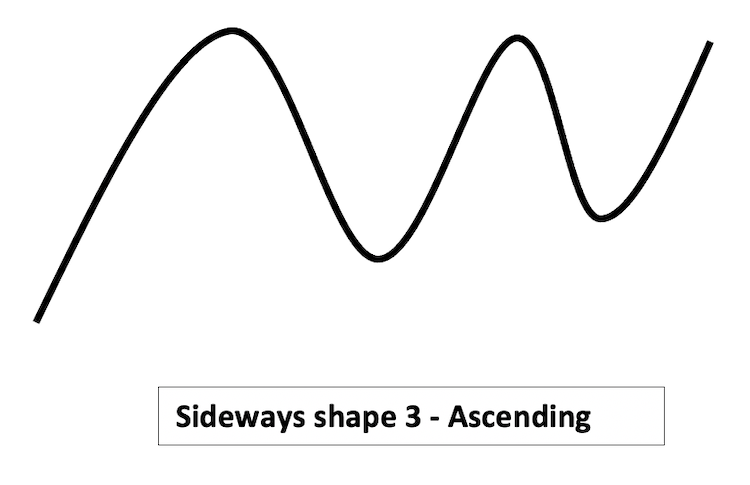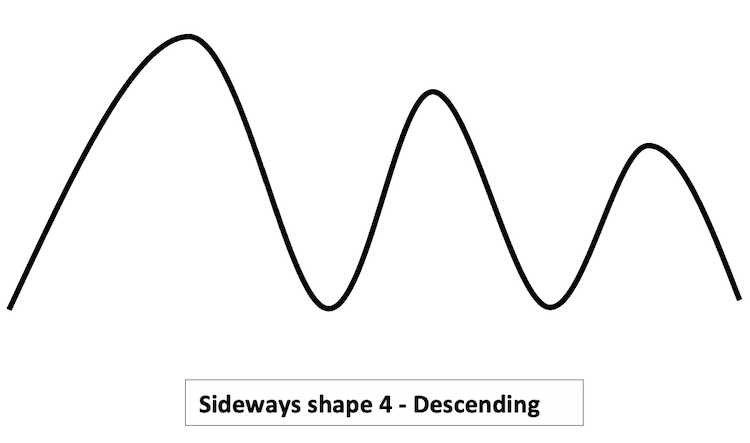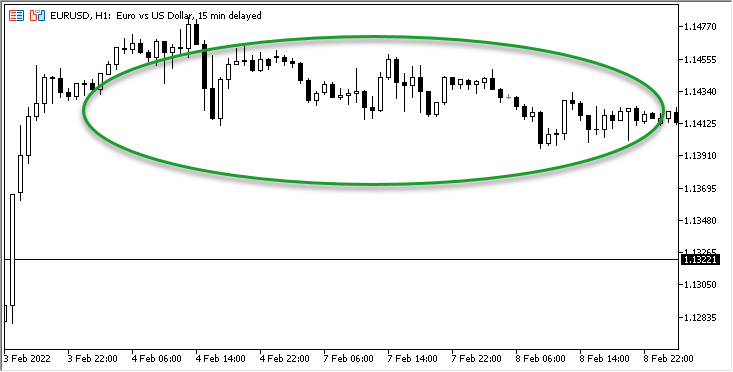• 策略二: 简单 ADX 系统 - 上行趋势:

ADX 值 > 25 且当前 ADX 值 > 前一个 ADX 值 --> 当 +DI 值 > -DI 值 = 做多

• 策略三: 简单 ADX 系统 - 下行趋势:

ADX 值 > 25 且当前 ADX 值 > 前一个 ADX 值 --> 当 +DI 值 < -DI 值 = 做空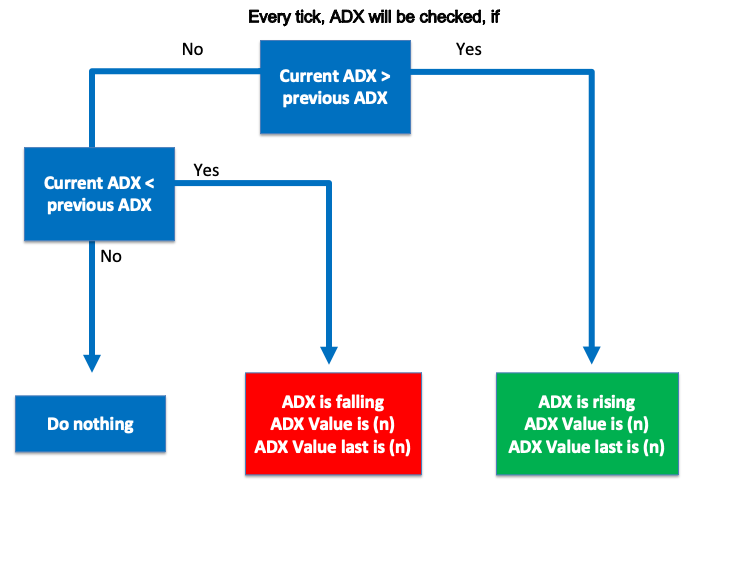• 策略二: 简单 ADX 系统 - 上行趋势: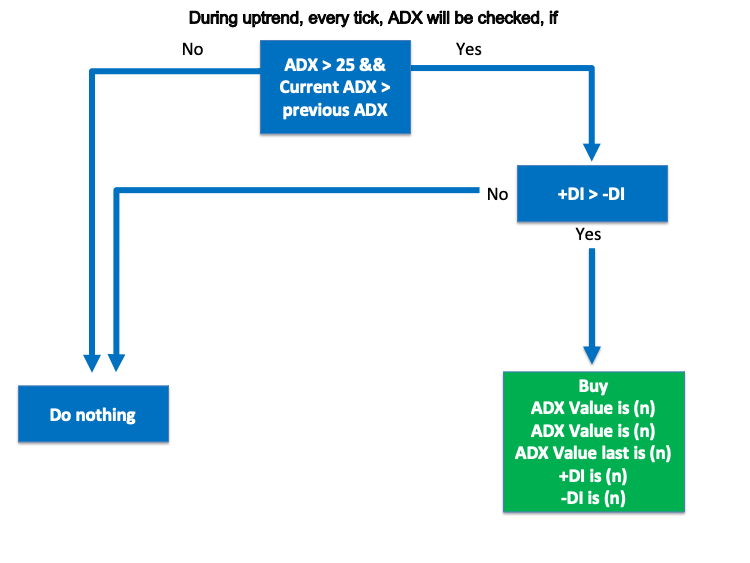• 策略三: 简单 ADX 系统 - 下行趋势: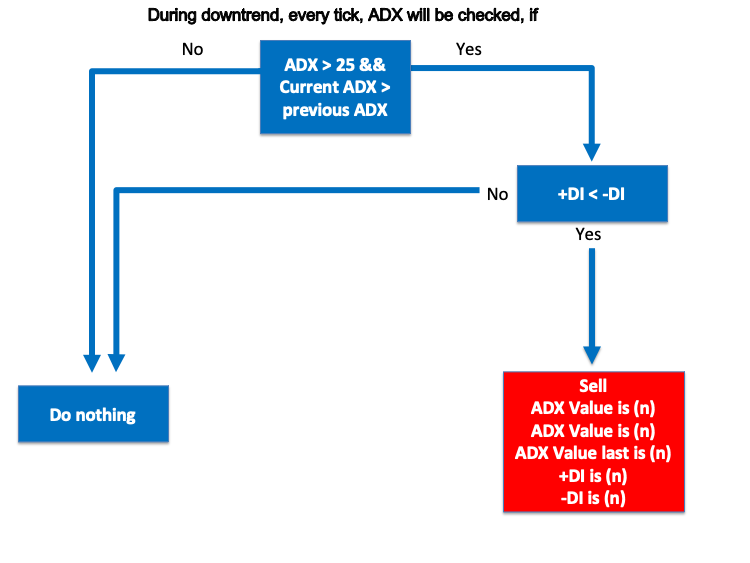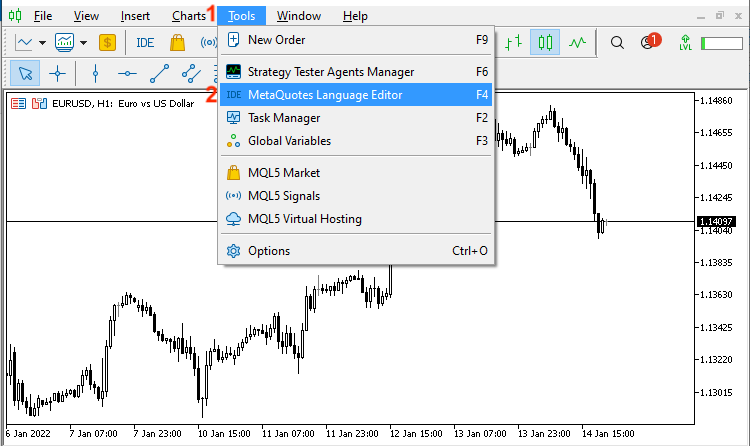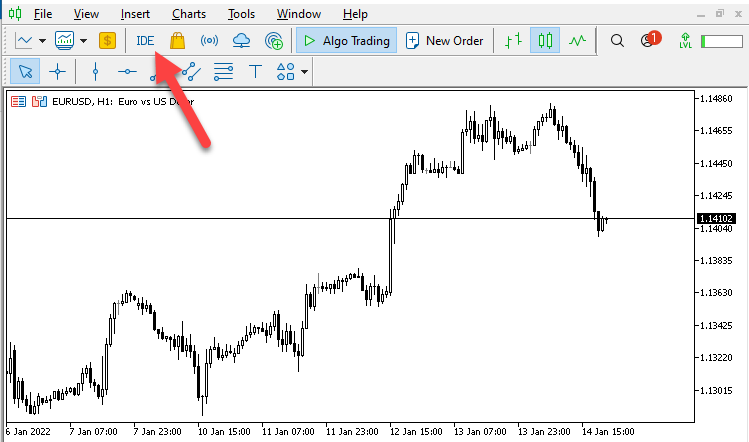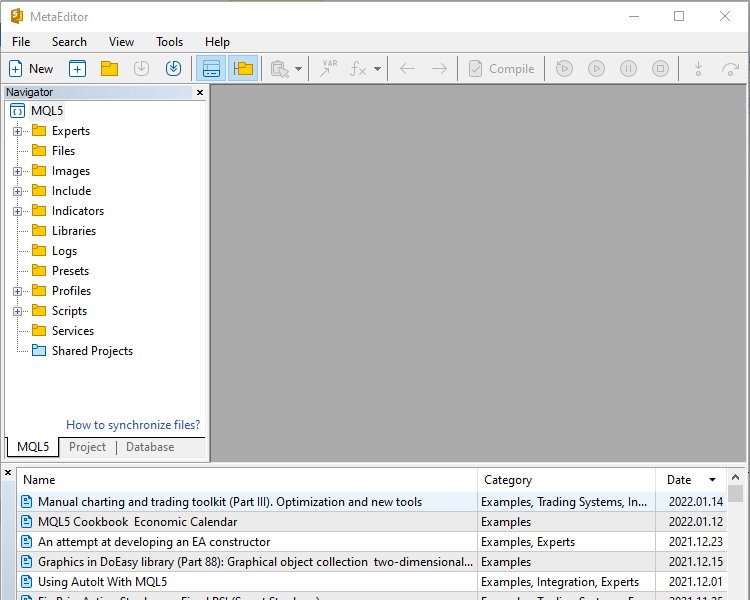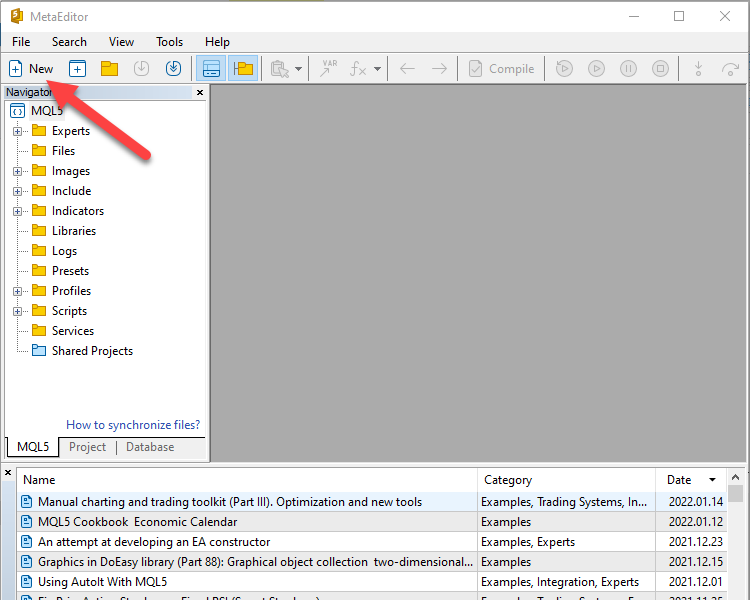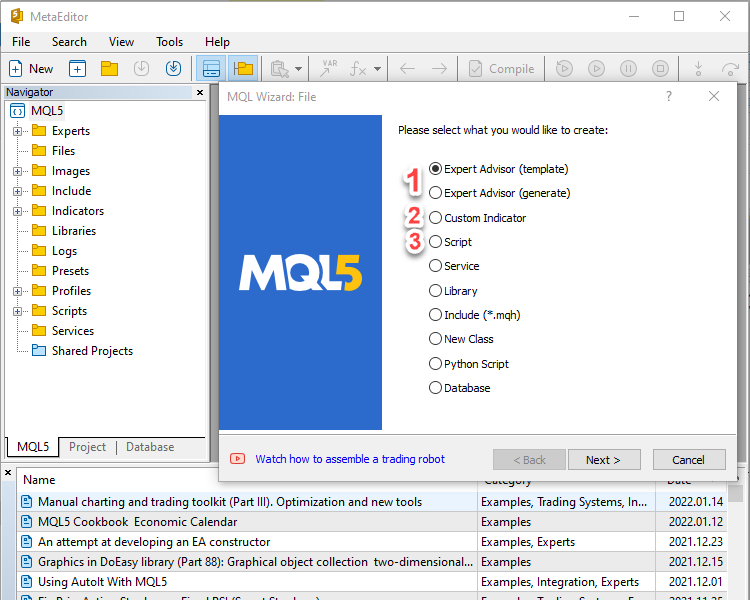2. Custom Indicator: 这种类型的程序代码可帮助我们读取图表，来改进交易决策。
3. Script: 在执行后仅能执行一次任务的程序。

• 创建一个价格数组，我们将使用 “double” 函数，该函数用分数部分表示数值：
`double PriceArray[];`
• 为所创建的价格数组里的当前数据设定排列顺序，我们将使用 “ArraySetAsSeries” 函数，它在成功时返回 true，在失败时返回 false（bool — 布尔值）。 其所需参数为：

### `ArraySetAsSeries(PriceArray,true);`

• _Symbol: 当前品种
• _Period: 当前时间帧
• 14: 计算周期
`int ADXDef = iADX(_Symbol,_Period,14);`
• 依据创建的 ADX 指标填充数据，我们将使用 “CopyBuffer” 函数，该函数返回已复制的数据数量，或在出现错误时返回 -1。 其参数为:
indicator 句柄，buffer_num 指标缓存区编号，start_time (数据和时间的起始时间)，stop_time (数据和时间的终止时间)，和 buffer[] (目标数组)。
`CopyBuffer(ADXDef,0,0,3,PriceArray);`
value 是双精度类型值 (规格化数字)，和整数型小数位 (小数点后面的位数)
`double ADXValue=NormalizeDouble(PriceArray,2);`
• 显示含有当前 ADX 值的注释，我们将使用 “Comment” 函数，其参数为：

`Comment("ADX Value is ", ADXValue);`

```//+------------------------------------------------------------------+
//|                                  Copyright 2022, MetaQuotes Ltd. |
//|                                             https://www.mql5.com |
//+------------------------------------------------------------------+
#property version   "1.00"
//+------------------------------------------------------------------+
void OnTick()
{
//creating an array for prices
double PriceArray[];

//sort price array from current data
ArraySetAsSeries(PriceArray,true);

//Filling data according to created ADX

//Getting value of current data

}
//+------------------------------------------------------------------+```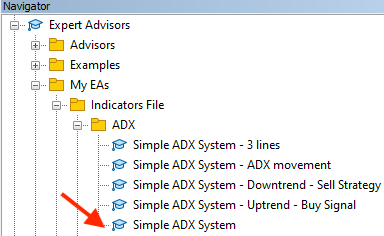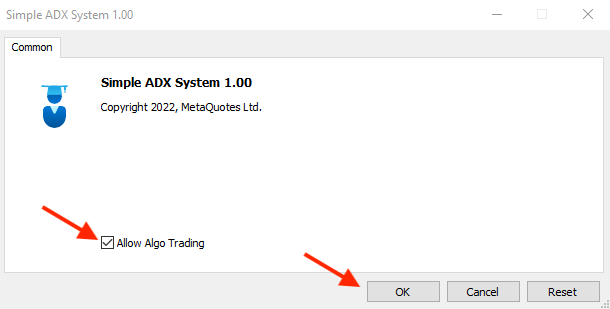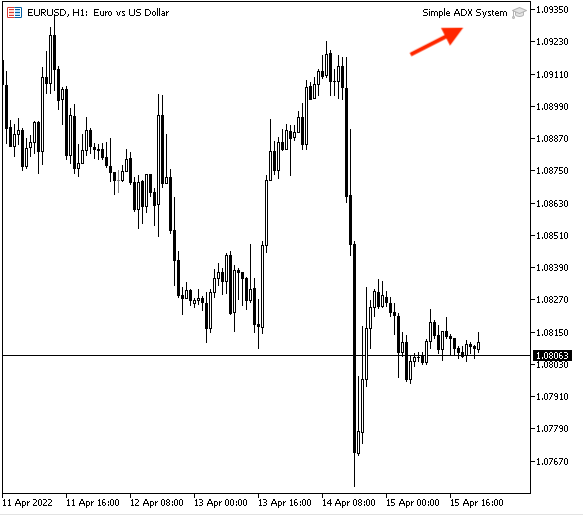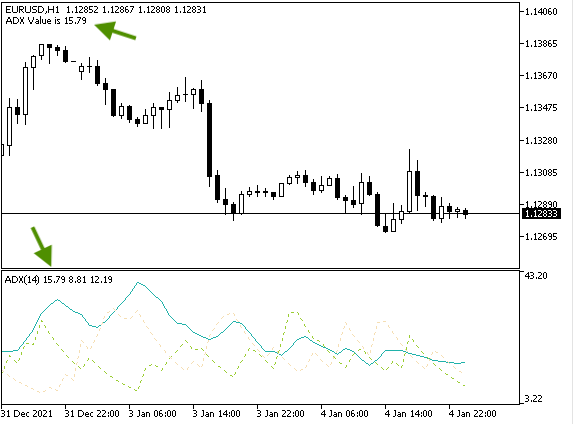• 为每个数值（ADX 值、+DI 和 -DI）创建价格数组，并用 “double” 类型为其创建变量：
• PDIArray: 用于 +DI 值
• NDIArray: 用于 -DI 值
```double ADXArray[];
double PDIArray[];
double NDIArray[];```
• 使用 “ArraySetAsSeries” 函数为当前数据中的每个价格数组设置排列顺序：
```ArraySetAsSeries(ADXArray,true);
ArraySetAsSeries(PDIArray,true);
ArraySetAsSeries(NDIArray,true);```

`int ADXDef = iADX(_Symbol,_Period,14);`
• 使用 “CopyBuffer” 函数依据根据识别的 ADX 填充数据：
```CopyBuffer(ADXDef,0,0,3,ADXArray);
• 获取当前的数值，使用 “double” 类型为每个值创建变量后，我们将使用 “NormalizeDouble: 函数：
```double ADXValue=NormalizeDouble(ADXArray,2);
double PDIValue=NormalizeDouble(PDIArray,2);
double NDIValue=NormalizeDouble(NDIArray,2);```
• 使用 “Comment” 函数在新的一行中显示每个值：
```Comment("ADX Value is ", ADXValue,"\n""+DI Value is ",
PDIValue,"\n""-DI Value is ", NDIValue);```

```//+------------------------------------------------------------------+
//|                                  Simple ADX System - 3 lines.mq5 |
//|                                  Copyright 2022, MetaQuotes Ltd. |
//|                                             https://www.mql5.com |
//+------------------------------------------------------------------+
#property version   "1.00"
//+------------------------------------------------------------------+
void OnTick()
{
//creating an array for prices
double PDIArray[];
double NDIArray[];

//sort price array from current data
ArraySetAsSeries(PDIArray,true);
ArraySetAsSeries(NDIArray,true);

//Filling data according to created ADX

//Getting value of current data
double PDIValue=NormalizeDouble(PDIArray,2);
double NDIValue=NormalizeDouble(NDIArray,2);

PDIValue,"\n""-DI Value is ", NDIValue);
}
//+------------------------------------------------------------------+```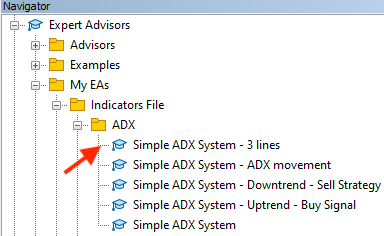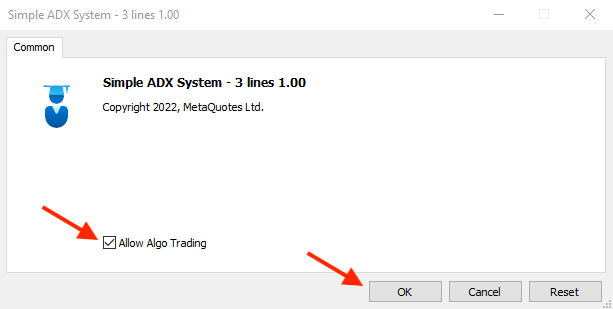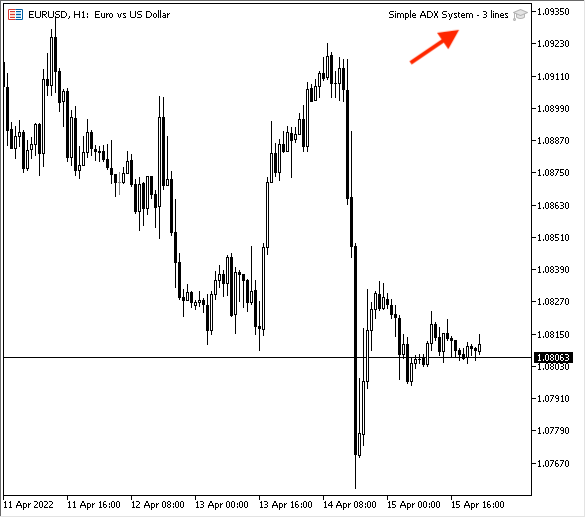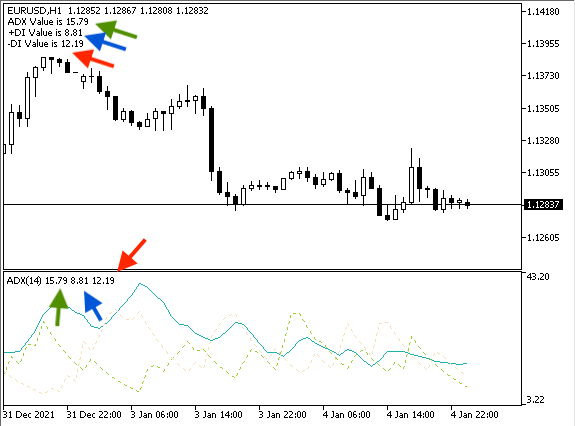```double ADXArray0[];

• 价格数组排列顺序从当前数据开始：
```ArraySetAsSeries(ADXArray0,true);

`int ADXDef = iADX(_Symbol,_Period,14);`
```CopyBuffer(ADXDef,0,0,3,ADXArray0);
• 创建变量后获取其值：
```double ADXValue=NormalizeDouble(ADXArray0,2);
```if (ADXValue>ADXValuelast)
{
}

{
}
```

```//+------------------------------------------------------------------+
//|                                  Copyright 2022, MetaQuotes Ltd. |
//|                                             https://www.mql5.com |
//+------------------------------------------------------------------+
#property version   "1.00"
//+------------------------------------------------------------------+
void OnTick()
{
//creating an array for prices

//sort price array from current data

//Filling data according to created ADX

//Getting value of current data

{
}

{
}
}
//+------------------------------------------------------------------+```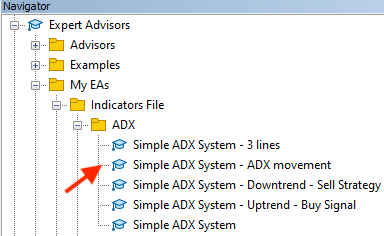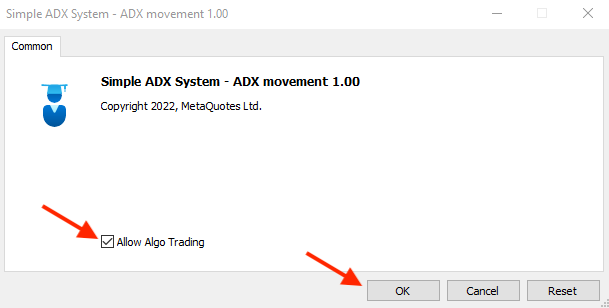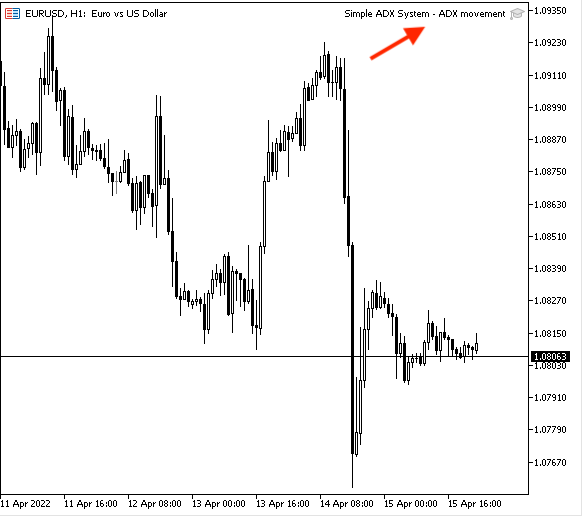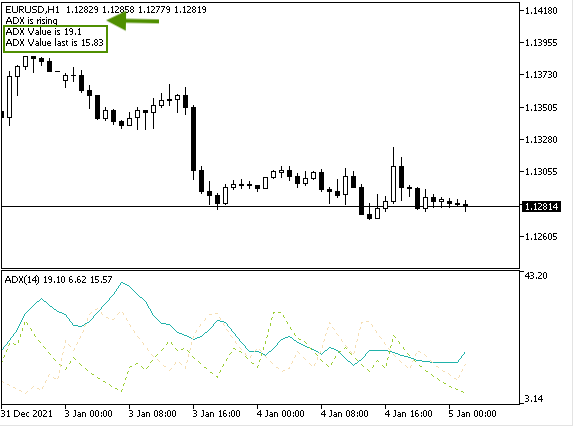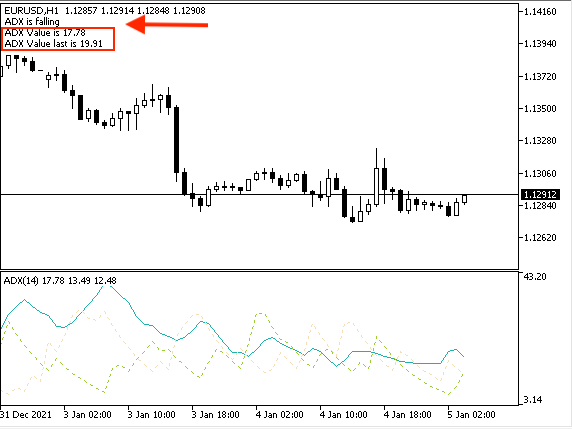• 策略二: 简单 ADX 系统 - 上行趋势:

ADX 值 > 25 且当前 ADX 值 > 前一个 ADX 值 --> 当 +DI 值 > -DI 值 = 做多

• 创建变量 "signal" 并赋值为空，因为会在之后再计算其值，我们将使用 “string” 类型：
`string signal="";`
• PDIArray: 用于 +DI 值
• NDIArray: 用于 -DI 值
```double ADXArray0[];
double PDIArray[];
double NDIArray[];```
• 价格数组排列顺序从当前数据开始：
```ArraySetAsSeries(ADXArray0,true);
ArraySetAsSeries(PDIArray,true);
ArraySetAsSeries(NDIArray,true);```
`int ADXDef = iADX(_Symbol,_Period,14);`
```CopyBuffer(ADXDef,0,0,3,ADXArray0);
• 获取当前数据值：
```double ADXValue=NormalizeDouble(ADXArray0,2);
double PDIValue=NormalizeDouble(PDIArray,2);
double NDIValue=NormalizeDouble(NDIArray,2);```
• 设置上升趋势策略的条件：
```if (ADXValue>25&&ADXValue>ADXValueLast)
if(PDIValue>NDIValue)
{
}```
• 注释里显示做多信号和数值，每个都在一行中：
```Comment("Simple ADX System - Signal is ",signal,"\n""ADX Value is ", ADXValue,
"\n""-DI Value is ", NDIValue);```

```//+------------------------------------------------------------------+
//|                                  Copyright 2022, MetaQuotes Ltd. |
//|                                             https://www.mql5.com |
//+------------------------------------------------------------------+
#property version   "1.00"
//+------------------------------------------------------------------+

void OnTick()
{

// creating a variable for signal
string signal="";

//creating an array for prices
double PDIArray[];
double NDIArray[];

//identify ADX, positive DI, negative DI

//sort price array from current data
ArraySetAsSeries(PDIArray,true);
ArraySetAsSeries(NDIArray,true);

//Filling data according to created ADX

//Getting value of current data
double PDIValue=NormalizeDouble(PDIArray,2);
double NDIValue=NormalizeDouble(NDIArray,2);

if(PDIValue>NDIValue)
{
}

"\n""-DI Value is ", NDIValue);
}
//+------------------------------------------------------------------+```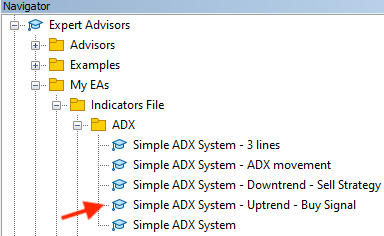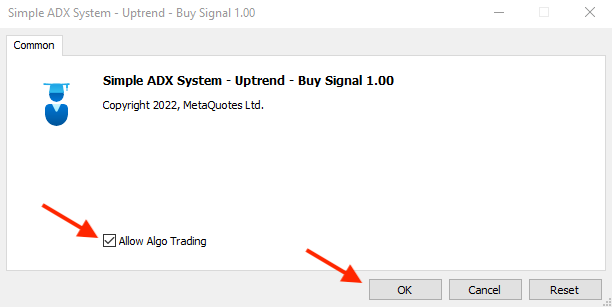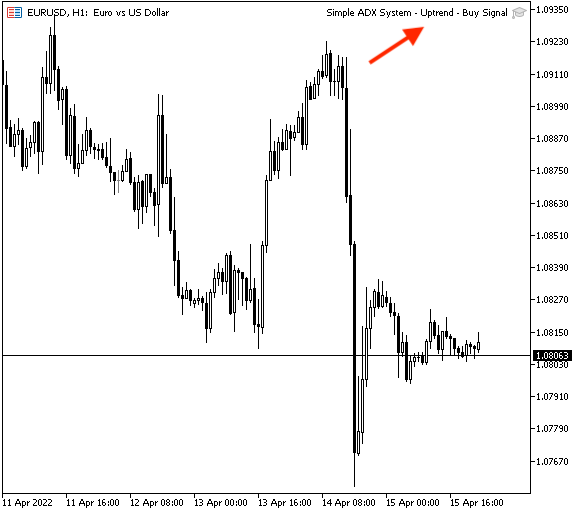• 做多信号。
• +DI 值出现在新的一行。
• -DI 值出现在新的一行。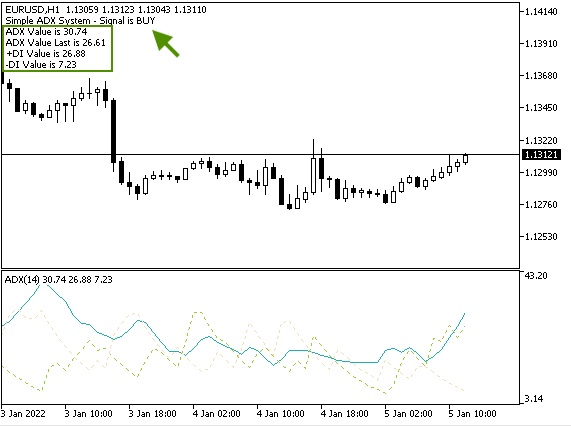• 策略三: 简单 ADX 系统 - 下行趋势:

ADX 值 > 25 且当前 ADX 值 > 前一个 ADX 值 --> 当 +DI 值 < -DI 值 = 做空

```//+------------------------------------------------------------------+
//|                Simple ADX System - Downtrend - Sell Strategy.mq5 |
//|                                  Copyright 2022, MetaQuotes Ltd. |
//|                                             https://www.mql5.com |
//+------------------------------------------------------------------+
#property version   "1.00"
//+------------------------------------------------------------------+
void OnTick()
{

// creating a variable for signal
string signal="";

//creating an array for prices
double PDIArray[];
double NDIArray[];

//identify ADX, positive DI, negative DI

//sort price array from current data
ArraySetAsSeries(PDIArray,true);
ArraySetAsSeries(NDIArray,true);

//Filling data according to created ADX

//Getting value of current data
double PDIValue=NormalizeDouble(PDIArray,2);
double NDIValue=NormalizeDouble(NDIArray,2);

if(PDIValue<NDIValue)
{
signal = "SELL";
}

"\n""-DI Value is ", NDIValue);
}
//+------------------------------------------------------------------+```

• 下行策略的条件：
```    if (ADXValue>25&&ADXValue>ADXValueLast)
if(PDIValue<NDIValue)
{
signal = "SELL";
}```
• 依据信号显示注释：
```   Comment("Simple ADX System - Signal is ",signal,"\n""ADX Value is ", ADXValue,
"\n""-DI Value is ", NDIValue);```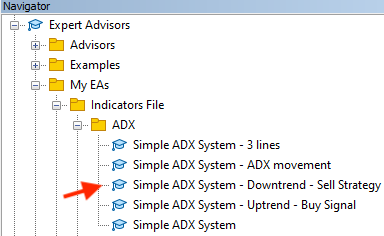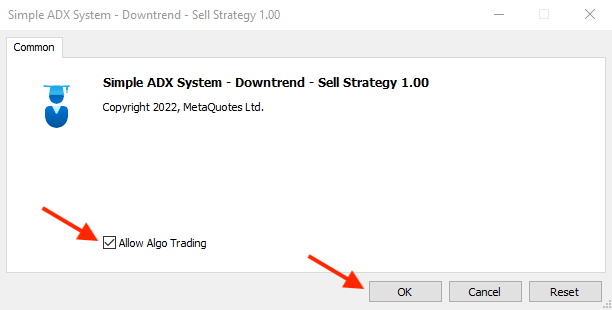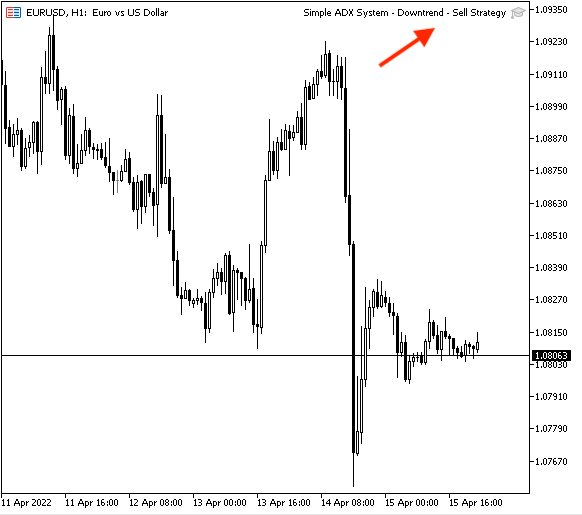• 做空信号。
• +DI 值出现在新的一行。
• -DI 值出现在新的一行。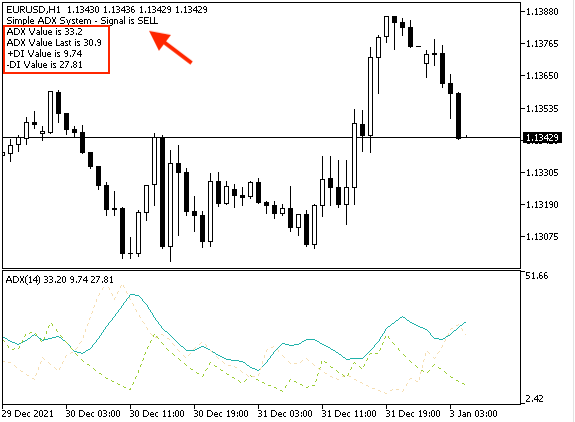### 结束语

#### 该作者的其他文章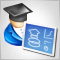从头开始开发智能交易系统（第 13 部分）：时序与交易（II）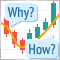学习如何基于 Stochastic 设计交易系统从头开始开发智能交易系统（第 14 部分）：添加价格成交量（II）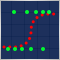数据科学与机器学习（第 02 部分）：逻辑回归